﻿ 航空发动机尾喷管热流固耦合分析 Thermal-Fluid-Solid Coupling Analysis of Aero-Engine Nozzle

Journal of Aerospace Science and Technology
Vol.04 No.04(2016), Article ID:19426,11 pages
10.12677/JAST.2016.44011

Thermal-Fluid-Solid Coupling Analysis of Aero-Engine Nozzle

Pei Luo, Min Zheng

College of Civil Aviation, Nanjing University of Aeronautics and Astronautics, Nanjing Jiangsu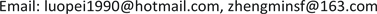Received: Dec. 9th, 2016; accepted: Dec. 26th, 2016; published: Dec. 29th, 2016ABSTRACT

The aero-engine nozzle works in the high temperature and high pressure environment, which is affected by the complex loads. The thermal load and the aerodynamic load are the main factors influencing the nozzle durability. Aiming at the problem of thermal-fluid-solid coupling for nozzle affected by high intensive pressure and high constant temperature, on the basis of finite element analysis, this paper applies ANSYS to build technology roadmap simulating one-way three fields coupling of an aircraft engine nozzle. Simultaneously, by means of analyzing main coupling characteristics, the results show the relationship among temperature field, flow field and the structure in the modal analysis, which has significant meaning in the design process of nozzle.

Keywords:One-Way Thermal-Fluid-Solid Coupling, Finite Element, Nozzle1. 引言

2. 热流固耦合方法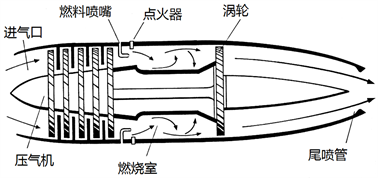Figure 1. Basic structure of turbojet engine

$\frac{\partial \rho }{\partial t}+\frac{\partial \left(\rho {v}_{x}\right)}{\partial x}+\frac{\partial \left(\rho {v}_{y}\right)}{\partial y}+\frac{\partial \left(\rho {v}_{z}\right)}{\partial z}=0$ (2-1)

$\nabla =i\frac{\partial }{\partial x}+j\frac{\partial }{\partial y}+k\frac{\partial }{\partial z}$ (2-2)

 (2-3)

$\left\{\begin{array}{l}\frac{\partial \left(\rho {v}_{x}\right)}{\partial t}+\nabla \cdot \left(\rho {v}_{x}\stackrel{⇀}{v}\right)=-\frac{\partial p}{\partial x}+\frac{\partial {\varsigma }_{xx}}{\partial x}+\frac{\partial {\varsigma }_{yx}}{\partial y}+\frac{\partial {\varsigma }_{zx}}{\partial z}+\rho {\alpha }_{x}\\ \frac{\partial \left(\rho {v}_{y}\right)}{\partial t}+\nabla \cdot \left(\rho {v}_{y}\stackrel{⇀}{v}\right)=-\frac{\partial p}{\partial y}+\frac{\partial {\varsigma }_{xy}}{\partial x}+\frac{\partial {\varsigma }_{yy}}{\partial y}+\frac{\partial {\varsigma }_{zy}}{\partial z}+\rho {\alpha }_{y}\\ \frac{\partial \left(\rho {v}_{z}\right)}{\partial t}+\nabla \cdot \left(\rho {v}_{z}\stackrel{⇀}{v}\right)=-\frac{\partial p}{\partial z}+\frac{\partial {\varsigma }_{xz}}{\partial x}+\frac{\partial {\varsigma }_{yz}}{\partial y}+\frac{\partial {\varsigma }_{zz}}{\partial z}+\rho {\alpha }_{z}\end{array}$ (2-4)

$\frac{\partial \left(\rho E\right)}{\partial t}+\nabla \cdot \left(\rho E\stackrel{⇀}{v}\right)=\nabla \cdot \left(k\nabla E\right)+S$ (2-5)

$M\frac{{\text{d}}^{2}x}{\text{d}{t}^{2}}+D\frac{\text{d}x}{\text{d}t}+Sx+\varsigma =0$ (2-6)Figure 2. One-way Thermal-Fluid-Structural coupling principle chart

3. 尾喷管模型和网格划分

4. 模态分析和耦合计算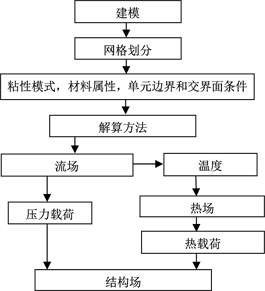Figure 3. Analysis flow chart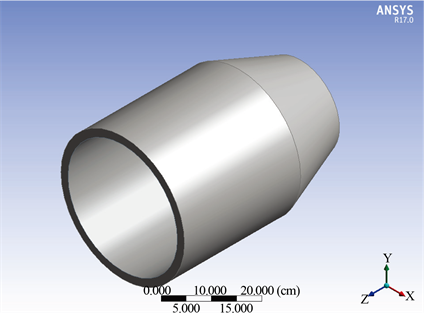Figure 4. Simplified nozzle model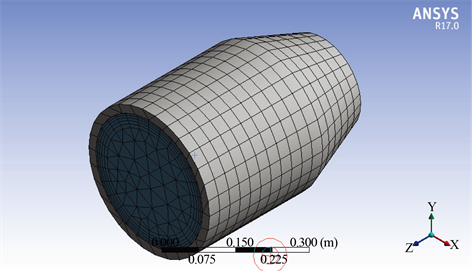Figure 5. Fluid field and structural meshing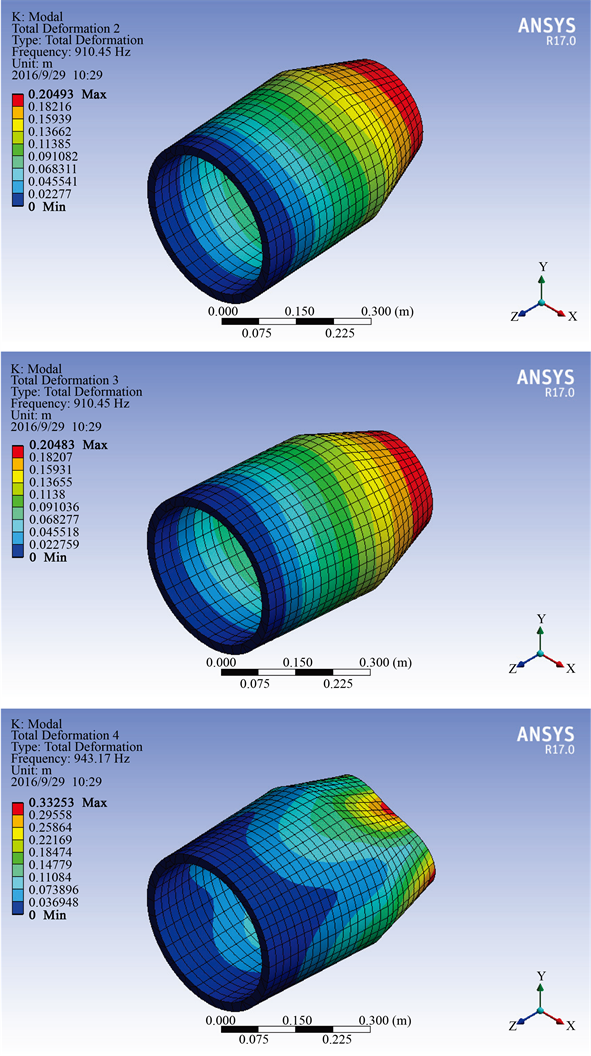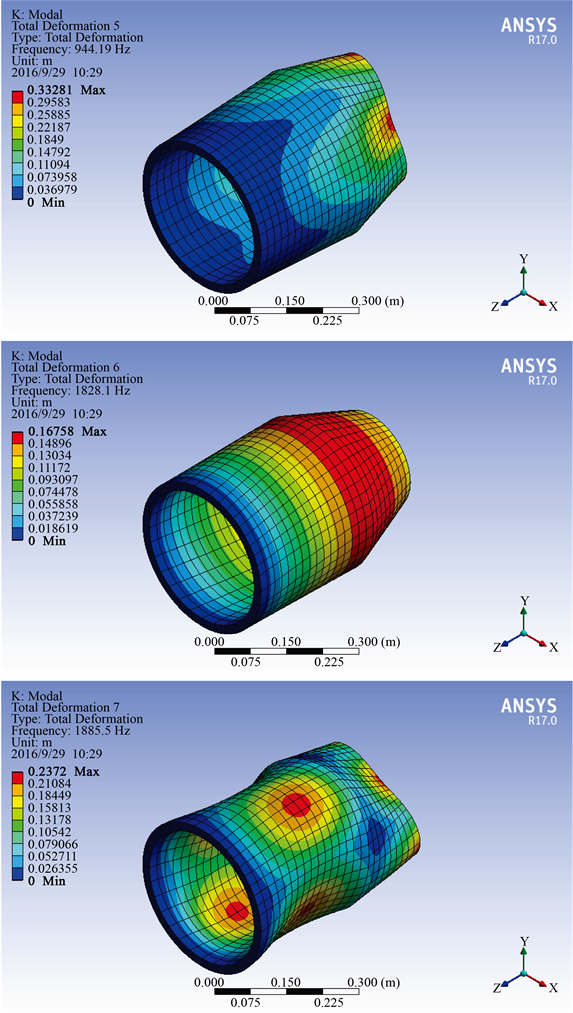Figure 6. Nozzle Six Vibration modes by no-pre-stress modal analysis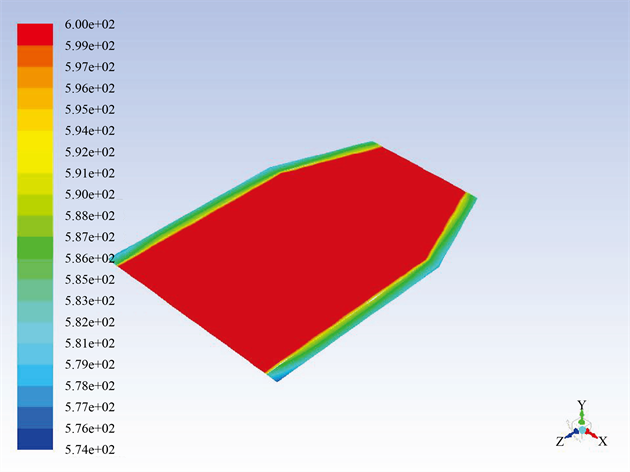Figure 7. Temperature distribution of fluid field and structural in the YZ plane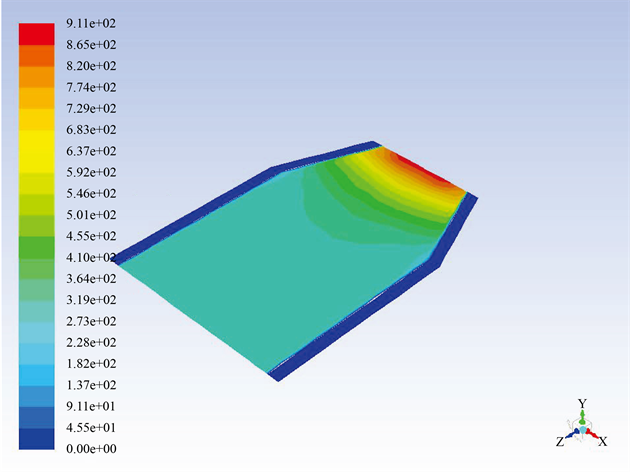Figure 8. Velocity distribution of fluid field and structural in the YZ plane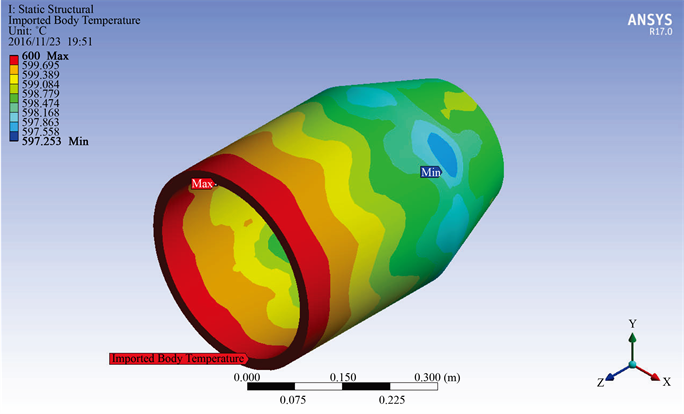Figure 9. Temperature load on the nozzle

5. 结论

1) 随着温度的升高，各阶振型的频率显著降低。由于温度的升高会导致材料密度降低，密度的降低进一步导致杨氏模量降低，从而使相同振型频率降低。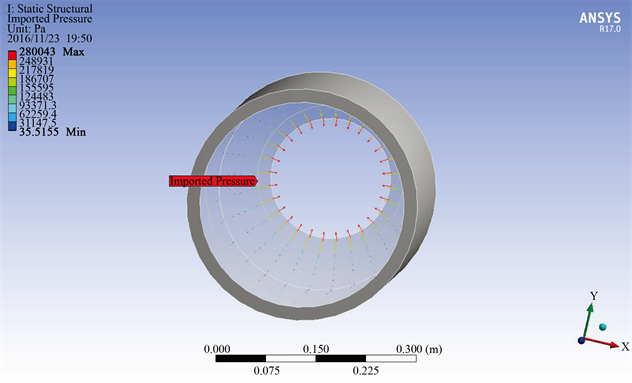Figure 10. Pressure load on the nozzle

2) 随着流速的升高，部分振型的频率轻微降低，部分振型频率变化不明显；阶数越高，其频率降低的幅度也越大。由于流场力对结构体的作用，造成结构形变，进而影响密度和杨氏模量，所以会有一定的影响。但总体言，流速对模态影响不明显。

 陈忠军, 刘熊. 燃气涡轮发动机基础[M].北京: 中国民航出版社, 2014.

 (2007) Archives of Pearson Scott Foresman. https://commons.wikimedia.org/wiki/File:Combustion_chamber_(PSF).png

 彭泽琰, 刘刚, 桂幸民, 等. 航空燃气轮机原理[M]. 北京: 国防工业出版社, 2008.

 廉筱纯, 吴虎. 航空发动机原理[M]. 西安: 西北工业大学出版社, 2005.

 Patankar, S.V. (1980) Numerical Heat Transfer and Fluid Flow. Hemisphere Publishing Corp, Carlsbad.

 Matarazzo, S. and Laget, H. (2011) Modelling of the Heat Transfer in a Gas Turbine Liner Combustor. Proceedings of the 7th Mediterranean Combustion Symposium, Chia Laguna, September, 56-68.

 Ji, Y.Z. (2003) The Status and Development of the Numerical Simulations of an Aero-Engine Combustion Chamber. Aero-Engine, 29, 25-29.

 高金海, 王建军, 马艳红, 洪杰. 基于热-流-固耦合方法火焰筒壁温三维数值模拟[J]. 北京航空航天大学学报, 2011(3): 981-985.

 董红, 闻雪友, 李名家, 贺星. 环管型燃烧室火焰筒壁温气热耦合数值模拟[J]. 航空动力学报, 2014(5): 1071-1078.

 杨万里, 陈国华, 王春发, 叶晓明. 内燃机燃烧室零件动态热应力研究[J]. 内燃机工程, 2002(3): 46-49.

 赵文胜. 轴向流中板结构流固耦合动力学研究[D]: [博士学位论文]. 武汉: 武汉大学, 2011.

 白洁. 发动机歧管式催化转化器热流耦合分析[D]: [硕士学位论文]. 武汉: 武汉理工大学, 2011.

 Pedlosky, J. (1987) Geophysical Fluid Dynamics. Springer, New York, 10-13. https://doi.org/10.1007/978-1-4612-4650-3

 Batchelor, G.K. (1967) An Introduction to Fluid Dynamics. Cambridge University Press, Cambridge.

 Wesseling, P. (2001) Principles of Computational Fluid Dynamics. Springer-Verlag, Berlin, Heidelberg. https://doi.org/10.1007/978-3-642-05146-3

 Olson, L.G. and Bathe, K. (1985) Analysis of Fluid-Structure Interactions. A Direct Symmetric Coupled Formulation Based on the Fluid Velocity Potential. Computers & Structures, 21, 21-32. https://doi.org/10.1016/0045-7949(85)90226-3

 周红梅, 于胜春, 王宏伟, 赵汝岩. 固体火箭发动机点火过程流固耦合仿真研究综述[J]. 推进技术, 2008(7): 54-57.

 谢超. 汽车空调离心通风机气动噪声双向流固耦合分析与优化[D]: [硕士学位论文]. 长沙: 湖南大学, 2014.

 邵学博. 基于ANSYS的机翼的流固耦合分析[D]: [硕士学位论文]. 哈尔滨: 哈尔滨工程大学, 2011.

 孟再强. 透平膨胀机叶轮流热固耦合数值模拟及分析[D]: [硕士学位论文]. 上海: 上海交通大学, 2013.

 吴云峰. 双向流固耦合两种计算方法的比较[D]: [硕士学位论文]. 天津: 天津大学, 2009.

 靳交通, 刘力根, 邓航, 冯学斌, 彭超义, 曾竟成. 基于有限差分法和有限元法的大型风电叶片刚度计算[J]. 机械设计与研究, 2012, 28(6): 83-85.

 Reddy, J.N. (2006) An Introduction to the Finite Element Method. McGraw-Hill Press, Columbus.

 (2016) ANSYS Release 17.0 User Documentation.

 胡海岩. 机械振动基础[M]. 北京: 北京航空航天大学出版社, 2005.